# SOHCAHTOA

SohCahToa is an acronym which helps you remember how to find the basic trig functions: sine, cosine and tangent.

## Use SohCahToa with a right triangle only!

To check if your triangle is the right triangle, look for the 90-degree angle. It’s the largest one in the triangle and it usually has a small square which indicates the right angle. (You can also think of it as a perfect corner of a room.)

If you don’t find the right angle, you can’t use the SohCahToa on your triangle.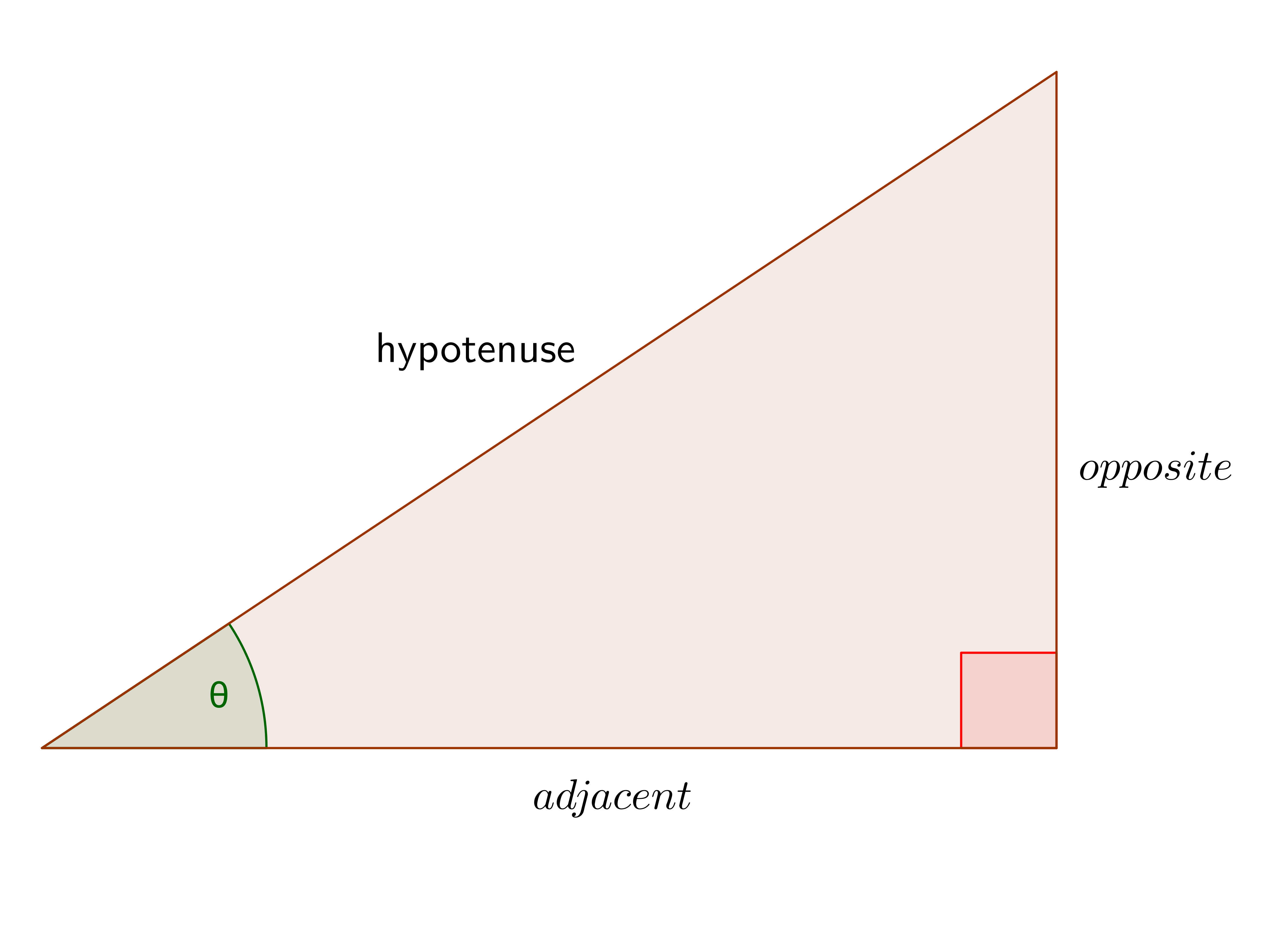## What does SohCahToa stand for?

SohCahToa stands for:

Sine
Opposite
Hypotenuse
Cosine
Hypotenuse
Tangent
Opposite

Hypotenuse, opposite, and adjacent are the sides of the right triangle. Before applying the SohCahToa, first identify the sides:

Hypotenuse – the side opposite to the right angle and the longest one in the triangle

Opposite –the side opposite from ? (the unknown angle)

Adjacent – the side next to ?; the angle ? is always placed between the hypotenuse and the adjacent side

Once you’ve spotted the right angle and all the sides, you can apply the SohCahToa to find the sine, cosine, and tangent of the acute angle ?:

sin? =

SOH: Sine is Opposite over Hypotenuse

cos? =

CAH: Cosine is Adjacent over Hypotenuse

tan? =

TOA: Tangent is Opposite over Hypotenuse

Lesson provided by Educator.com

## Example I

Find the values of sine, cosine and tangent of the angle ? from the triangle in the picture.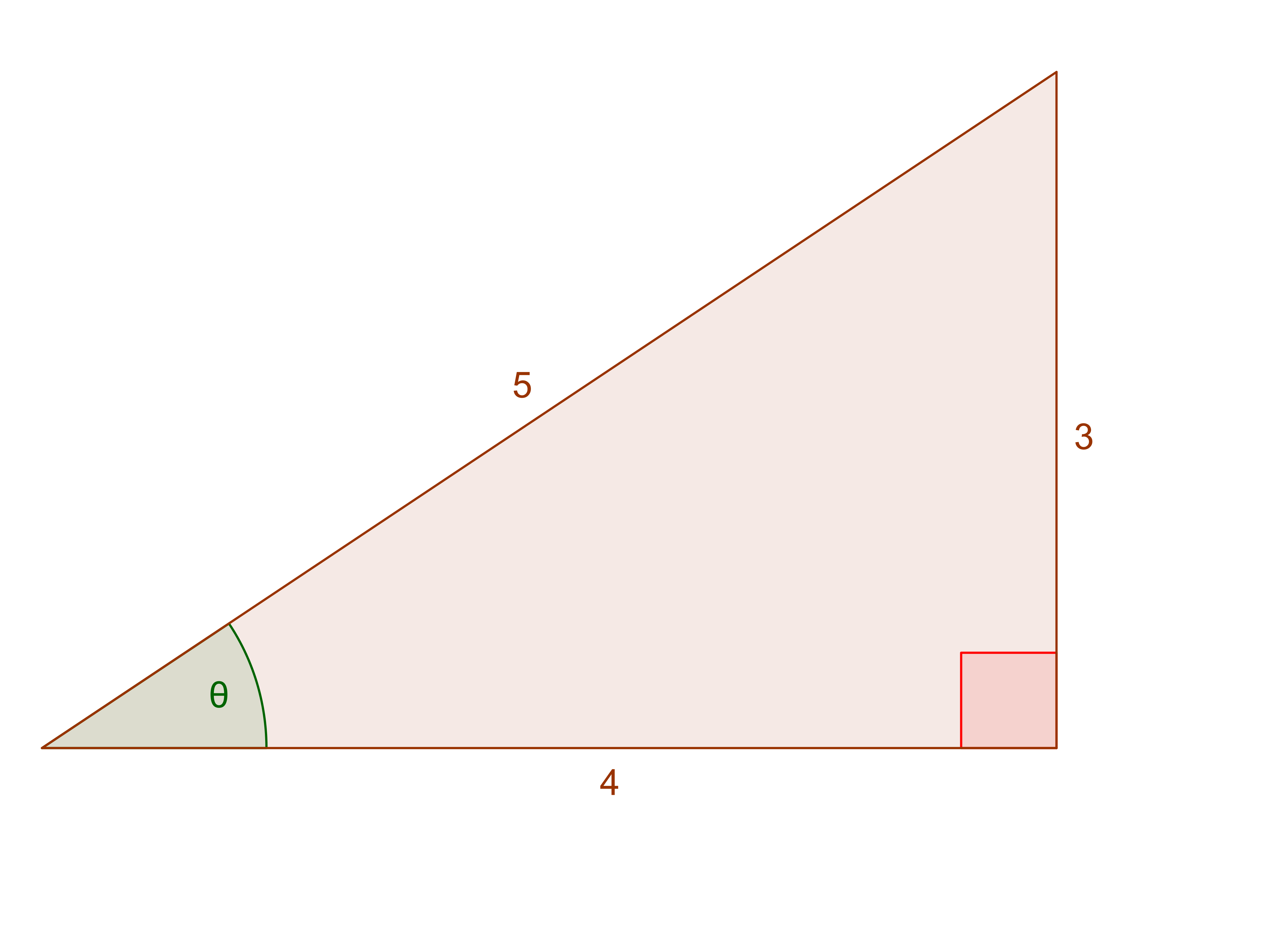Step 1: Identify the sides.

Hypotenuse is opposite to the right angle and the longest side. In the triangle above, the hypotenuse is the side with the length of 5.

Opposite is the side opposite to our angle ?. In the triangle above, the opposite is the side with the length of 3.

Adjacent is next to ?. In this case, it’s the one with the length of 4. Angle ? is sandwiched between the hypotenuse and the adjacent side.

Step 2: Write the SohCahToa on your paper.

This way you don’t get confused and writing it down every time will also help you memorize it.

Step 3: Apply the SohCahToa.

Sine is given in the “Soh” part:

sin? =

Now plug in the values (opposite is 3 and hypotenuse is 5):

sin? =

Cosine is given in the “Cah” part:

cos? =

Tangent is given in the “Toa” part:

tan? =

We have just found the basic trig functions for the lower left-hand corner.

## Example II

Let’s find the trig functions of the angle in the other corner of the triangle.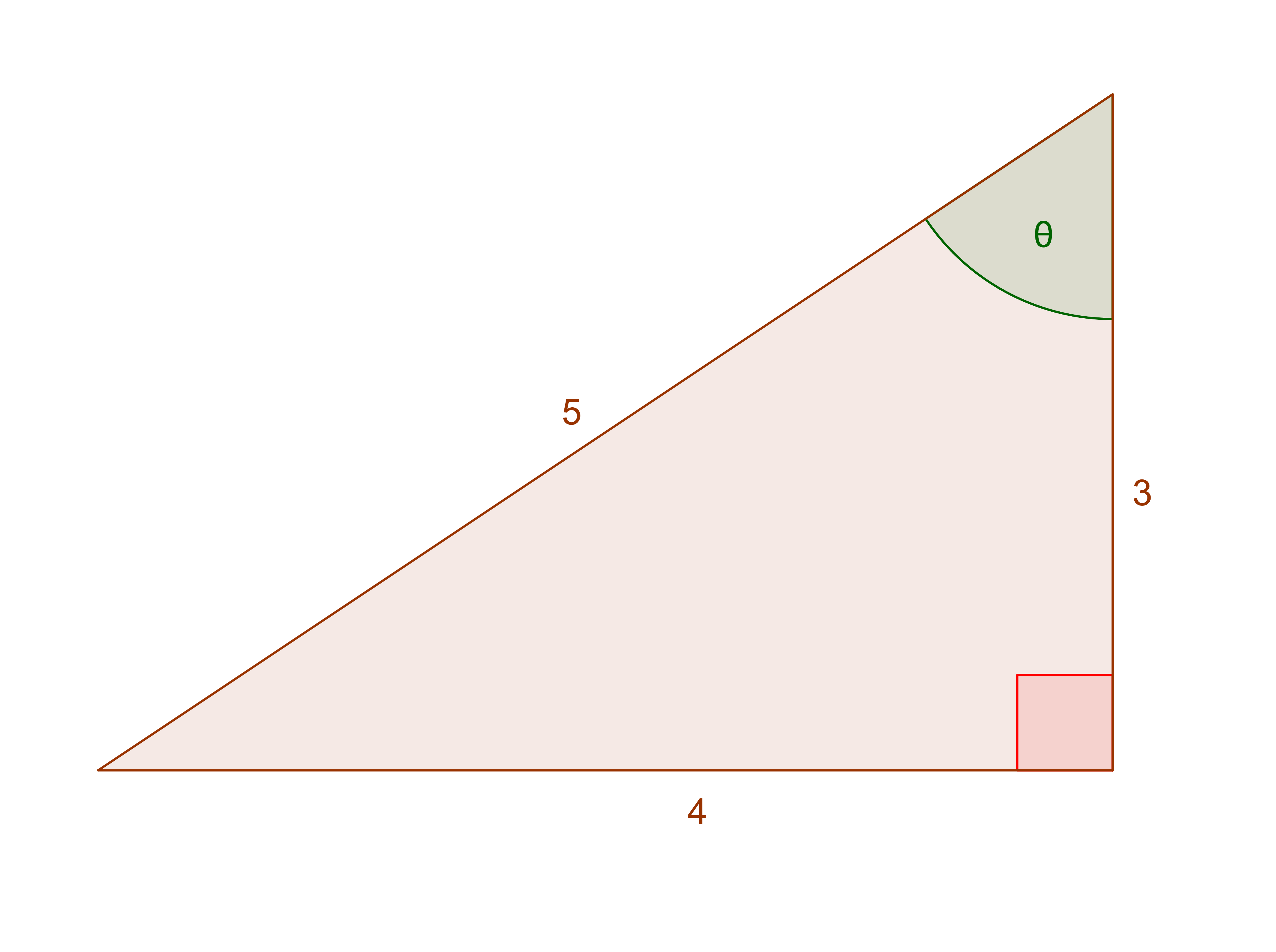Step 1: Identify the sides.

Hypotenuse is as same as in the previous example. It’s opposite to the right angle so it is 5.

But now our angle ? has changed, so we have to look carefully for the opposite and the adjacent side!

Opposite to ? is now 4 and adjacent is 3. Remember that ? is always between the hypotenuse and the adjacent.

Step 2: Write the SohCahToa on your paper, so that you have something to refer to.

Step 3: Apply the SohCahToa.

Sine is given in the “Soh” part:

sin? =

Cosine is given in the “Cah” part:

cos? =

Tangent is given in the “Toa” part:

tan? =

## Example III

Find the sine, cosine, and tangent of the angle ? in the following triangle:

This one might seem confusing at first, but don’t worry! If you look closely you’ll see that this is just a regular right triangle, only turned upside down. We can still apply the steps we did in the first two examples.

Step 1: Identify the sides.

Now you see how important this step is because you won’t always have your triangle turned exactly in the way you are used to.

Hypotenuse is opposite to the right angle so it’s 25.

Opposite is the side opposite to our angle ? so it’s 7.

Adjacent is next to ? so it’s 24. (Angle ? is between 25 and 24 – the hypotenuse and the adjacent.)

Step 2: Write the SohCahToa on your paper.

Step 3: Apply the SohCahToa.

We get the sine from the “Soh” part:

sin? =

We get the cosine from the “Cah” part:

cos? =

And we get the tangent from the “Toa” part:

tan? =

Be careful!

Remember that angle ? is always an acute angle. You can’t apply the SohCahToa on the right angle. If you tried this, you’ll get stuck on identifying the sides (hypotenuse and opposite would be the same). To find the trig functions of the 90-degree angle, you can use the unit circle.

## Example IV: How to find the measure of angle ??

In the previous examples we have found the sine of ?, cosine of ? and tangent of ?. But what is the measure of the angle ? alone?

Let’s say you are given the hypotenuse and the opposite side. We need to find the measure of angle ?.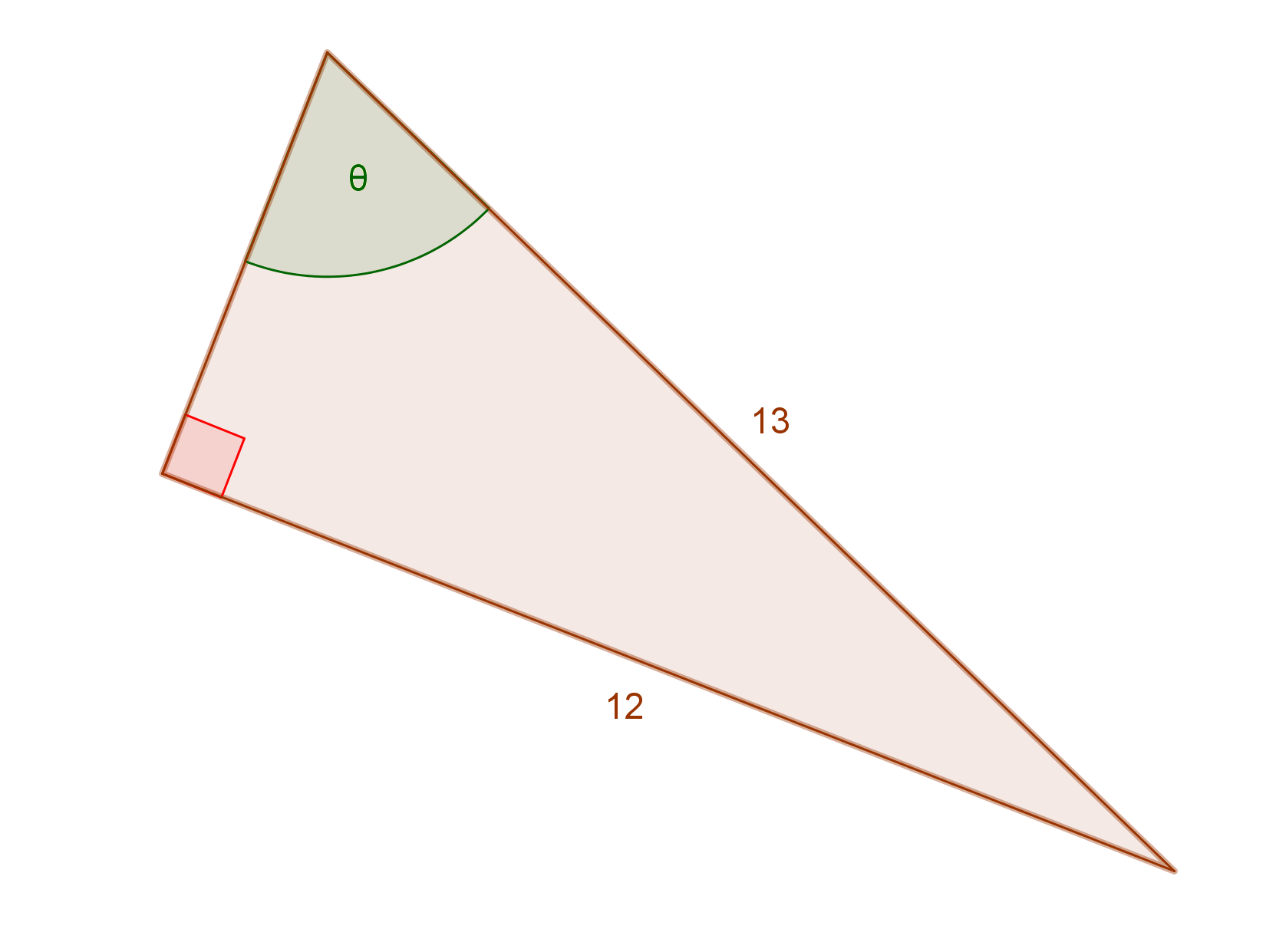Step 1 is to identify the sides. Hypotenuse is opposite to the right angle so hypotenuse is 13, while opposite is 12.

Step 2 is to write SohCahToa. We are given opposite (o) and hypotenuse (h), so let’s look for “oh” in the acronym we’ve just written: they appear with the sine (Soh), so we’ll use the sine to find our angle.

sin? =

sin? =

Now divide 12 and 13 using your calculator. Once you do that, you’ll get 0.923 approximately.

sin? = 0.923

The next step is to use the inverse function to get the measure of our angle. Using your calculator, find the sine inverse of 0.92:

And voila! Our angle is approximately 67°.

## Example V: How to find the length of a side?

If you are already given the angle and one side of your right triangle, you can easily find the length of a missing side.

Let’s say you are given angle ?=40° and one side with length 3. We need to find the length of x.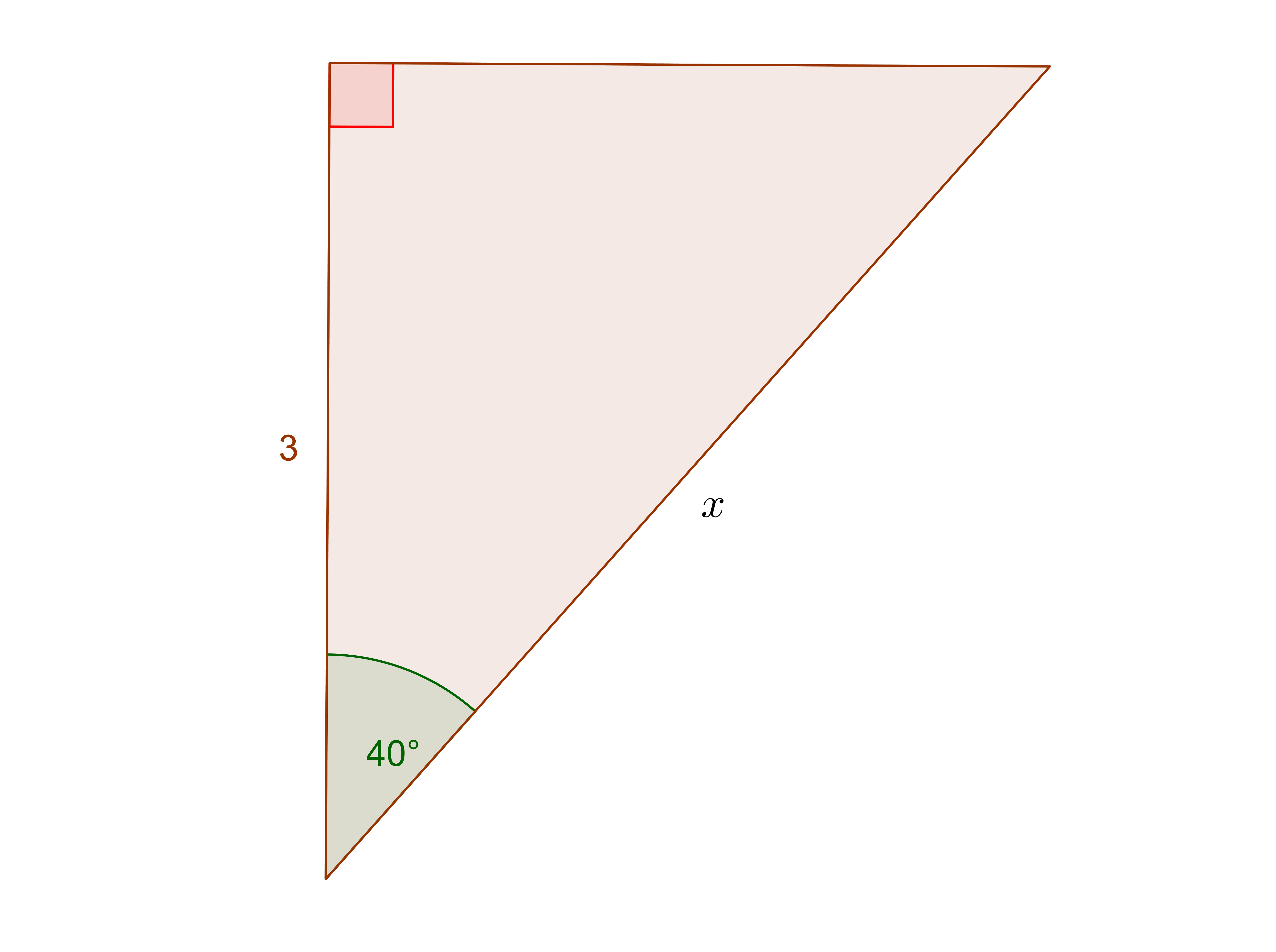Let’s first identify the sides. Hypotenuse is opposite to the right angle so hypotenuse is x. Side 3 is next to our angle ?=40° so it is the adjacent side.

Now let’s write SohCahToa and look for “ah” since we have adjacent and hypotenuse involved in our problem. The “ah” appears in “Cah” so we’ll use the cosine to determine the length of x.

cos40° =

cos40° =

Use the calculator to find the cos40° (it’s 0.766 approximately) and multiply both sides with x:

Now divide both sides with 0.766:

The length of the hypotenuse is 3.92.

Lesson provided by Educator.com

## Practice

Which trigonometric ratio would you use to determine the value of x? Justify your choice.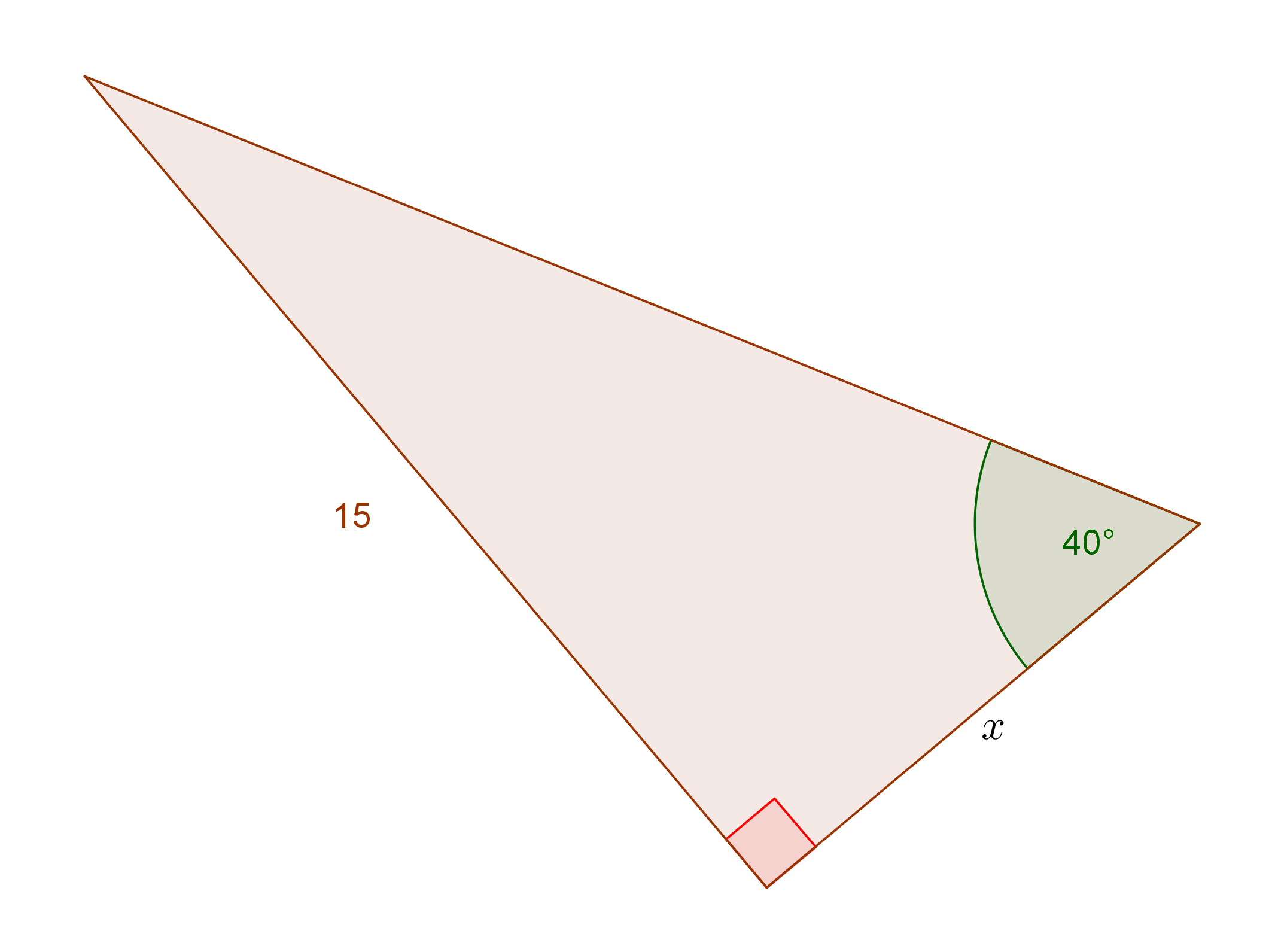We are given the angle ?=40° and its opposite side. The unknown side x is adjacent to ?. Since we have opposite and adjacent, we look for “oa” in SohCahToa. It appears with the tangent (Toa) so we’ll use:

tan? =

## Did this help?

[box type=”success” align=”” class=”” width=””]You can check out our other article on how to use SohCahToa to solve the Unit Circle and learn more topics in Trigonometry + 100 other subjects at Educator.com, the best place to get in-depth and instant homework help online.[/box]

SPECIAL OFFER!

SUCCESS8